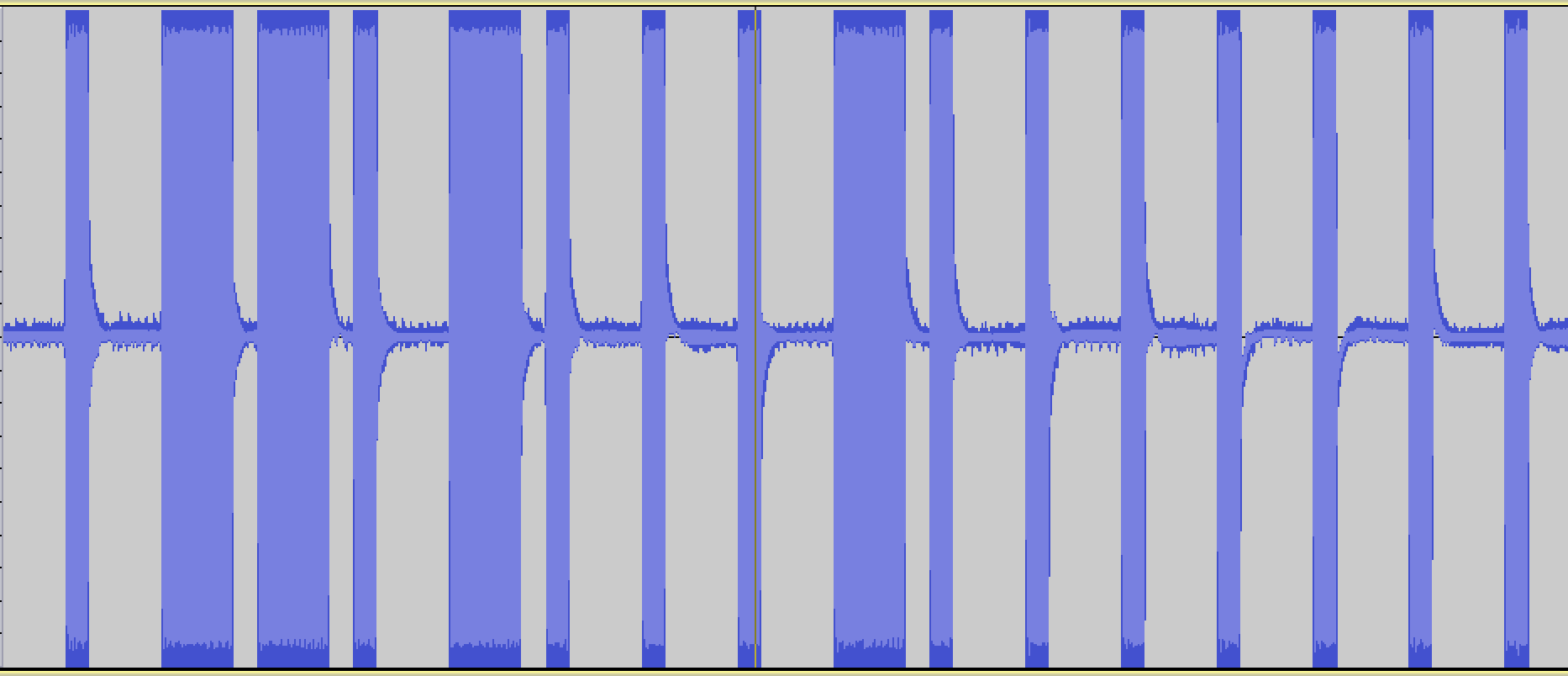## Programming, Hacking, Math, and Art

Recently, I was curious if I could generate a digital radio signal from scratch just using math, and use that signal to control this radio-controlled outlet that I have.

I want to be able to generate modulated digital signals such as this one...This is a binary signal coded with On-off keying (also known as Amplitude-shift keying).

I'm taking the long pulses to mean "1" and the short pulses to mean "0", so this signal is transmitting the digital code, "0110100010000000".

In :
# Imports and boilerplate to make graphs look better
%matplotlib inline
import matplotlib.pyplot as plt
import numpy as np
import scipy
import wave
from ...

# Fourier Transform Notes

The following are my notes from a talk I gave at OSCON 2013. Here is the Github source of these Jupyter/IPython notebooks: https://github.com/calebmadrigal/FourierTalkOSCON

# Sound Analysis with the Fourier Transform and Python

## Intro

1. Welcome
2. Format of talk
• Everything is in iPython Notebook (on Github)
• You don't need to take notes
• Please save questions for the end
3. Why this is interesting
• Sound processing is big - natural human-machine interfaces (e.g. Siri)
• Noise reduction, Compression, feature extraction (e.g. speech)
• Understanding our universe better (Superposition, Harmonics, Sound timbre)

## Overview

• The Nature of Waves
• The Fourier Transform
• Fast Fourier Transform (FFT) in Python
• Audio analysis

In :
%matplotlib inline
import matplotlib.pyplot as plt
import numpy as np
import ...